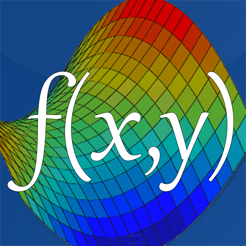## Capturas de tela

•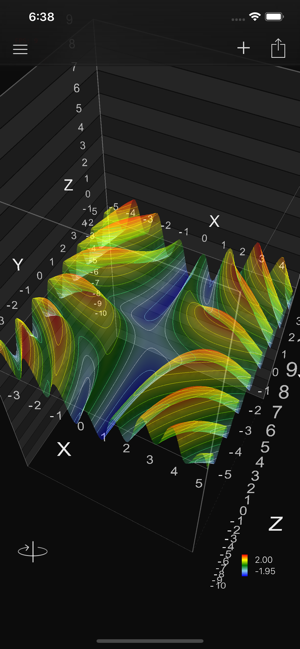•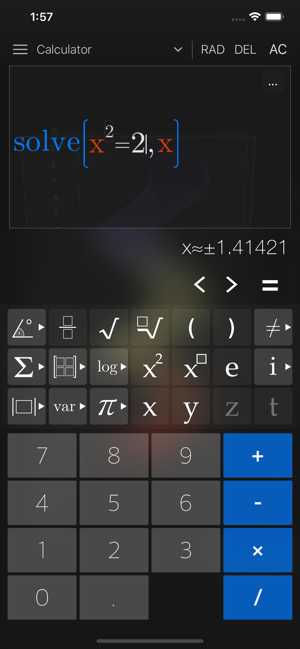•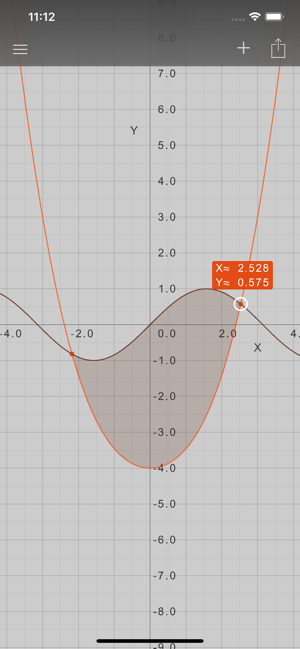•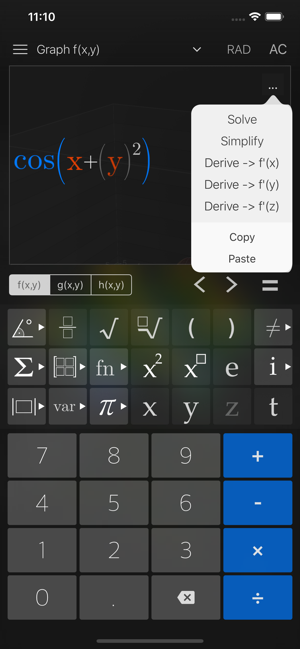•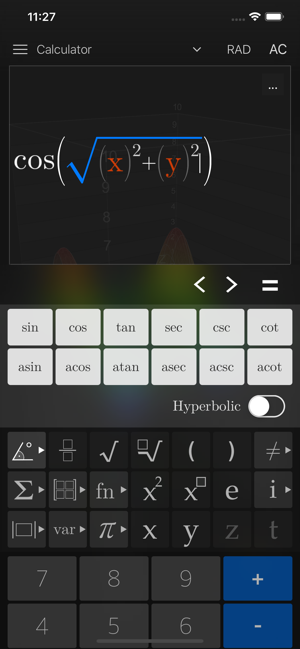•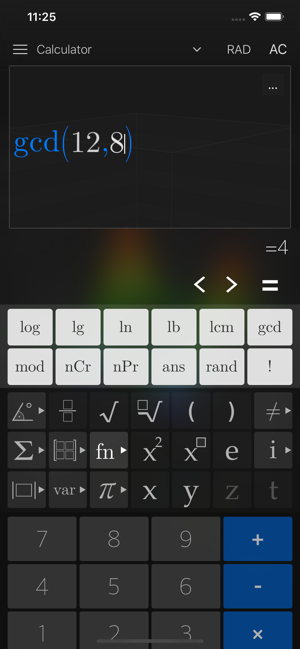•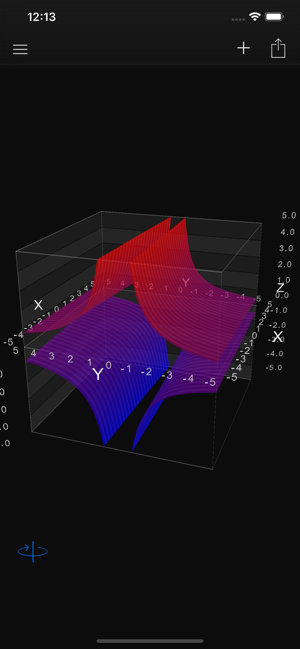•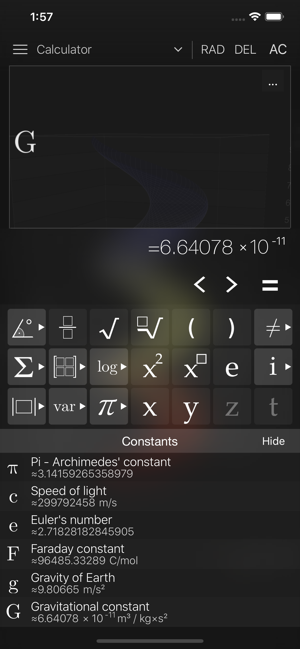•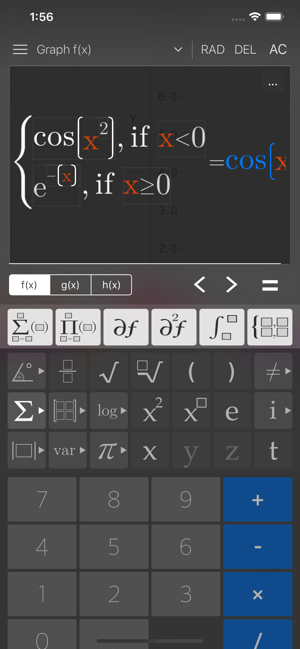•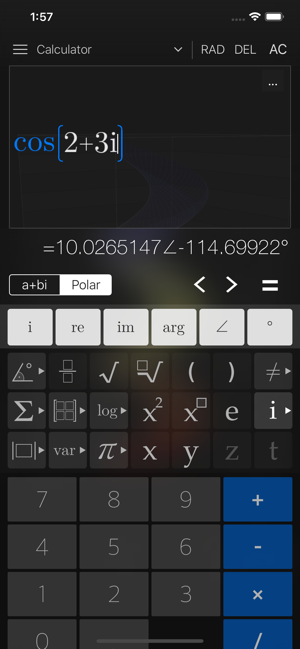## Descrição

Visual Math 4D is a graphical calculator that allows you to visualize and solve your mathematical equations. It supports various types of equations, such as polar, spherical, parametric and implicit equations, which can be visualized and animated in 2D and 3D. You can also plot and animate vector fields in 2D and 3D.

Features:
- solve equations
- plot cartesian functions with intersections
- plot polar- and spherical functions
- plot parametric equations in 2D and 3D
- plot complex functions (draw real part & imaginary part)
- plot vector fields in 2D and 3D
- plot implicit equations in 2D and 3D
- plot contour maps ins 2D and 3D
- support for complex numbers
- vectors & matrices
- truth- and value tables
- trigonometrical and hyperbolic functions
- piecewise-defined functions
- logarithmic functions
- logical & binary operators
- definite integral
- n-th derivations
- statistical functions (nPr, nCr, rand..)
- physical and mathematical constants with their units
- animate variables
- share content with other apps
- no network connection required
- and a lot more

The app is easy to use and helps students and engineers to visualize and solve their mathematical equations.

Versão 5.3

- draw intersection points and show their coordinates
- optimized expression rendering
- select and focus functions using gestures.
- new functions are supported, like modulo, fact, nPr, nCr, lcm, gcd, rand etc..
- support for the last result (ans)
- copy & paste your equations
- new device support

## Avaliações e opiniões

4.8 de 5
31 avaliações

31 avaliações

rfloc ,

### Muito bom

Eu gostei bastante

## Informações

Vendedor
Ronny Weidemann
Tamanho
117.2 MB
Categoria
Educação

Requer o iOS 10.0 ou posterior. Compatível com iPhone, iPad e iPod touch.

Idiomas
Classificação indicativa
4+
Preço
R\$ 7,90
Compras dentro do app
1. Coffee R\$ 3,90
2. Cappuccino R\$ 7,90
3. Sandwich R\$ 18,90

## Compatível com

•